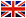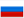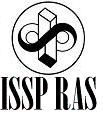Physics of Semiconductors

Prof., Dr.Sc. V.D.Kulakovskii

Annotation

The course focuses on the presentation of foundations of physics of semiconductors. Along with the traditional branches of physics semiconductors the course deals modern problems such as composite quasiparticles in low dimensional semiconductor nanostructures.

Program

1. Transport phenomena in semiconductors: electrical conductivity, Hall effect, the effect of the magnetic field and temperature gradient. The electron mean free time.
2. Elementary theory of galvanomagnetic phenomena (electrical conductivity tensor in a magnetic field, the Hall angle and Hall coefficient, magnetoresistance), mixed conductivity, experimental measurements of the conductivity and Hall effect.
3. Chemical bonds in the crystal lattices of semiconductors, electronic configuration of atoms, types of chemical bonds: ionic bond, covalent bond, van-der-Waals bond, crystals with mixed bonds, non-crystalline semiconductors.
4. Semiconductor properties and chemical bonding. Band gap, impurity levels, vacancies in the crystal.
5. Elementary band theory of semiconductors (perfect lattice). Key assumptions of band theory. The wave function of an electron in a periodic field. Brillouin zone, the energy bands.
6. The method of tightly bound electrons.
7. The dispersion of electrons and holes. Effective mass. Examples of the band structure of semiconductors.
8. The elements of band theory of semiconductors (semiconductors in external fields, imperfect crystals). The mean values of the velocity and acceleration of the electron, the electrons and holes in a magnetic field (the classical theory), the diamagnetic resonance.
9. The effective mass. The energy spectrum of electrons and holes in a magnetic field (quantum theory), the energy spectrum of electrons and holes in a constant electric field (quantum theory), shallow impurity levels.
10. Statistics of electrons and holes in semiconductors. Distribution of quantum states in the energy bands, Fermi-Dirac distribution, the effective density of states in the bands, the carrier concentration in the degenerate and nondegenerate semiconductors, the concentration of electrons and holes at the local levels.
11. Gibbs distribution. The Fermi level in intrinsic and doped semiconductors.
12. Phenomena in the contacts. Potential barriers, the current density, the Einstein relation, the conditions of equilibrium, thermionic work function, the contact potential difference.
13. The distribution of the electron density and potential in space-charge layer, the screening length, enriched and depleted layer. Rectification in contact metal – semiconductor, p-n-junction.
14. Nonequilibrium electrons and holes in semiconductors. Lifetime of nonequilibrium electrons and holes. The continuity equation. Photoconductivity. Quasi-Fermi levels.
15. The problems of justification of the band theory. The adiabatic approximation, the approximation of small oscillations, the method of self-consistent field.
16. Polarons, excitons, excitonic molecules, ionization of excitons, electron-hole plasma, electron-hole liquid.
17. Optical transitions in direct and indirect semiconductors. Optical band-to-band and excitonic absorption and emission.
18. Exciton polaritons in bulk semiconductors, exciton polaritons in semiconductor microcavities.
19. Bose-Einstein condensation of microcavity polaritons.

MAIN REFERENCES

1.VL Bonch-Bruevich S.G.Kalashnikov Semiconductor Physics, Nauka, 1977

2..I.Anselm Introduction to Semiconductor M., FML. 1962